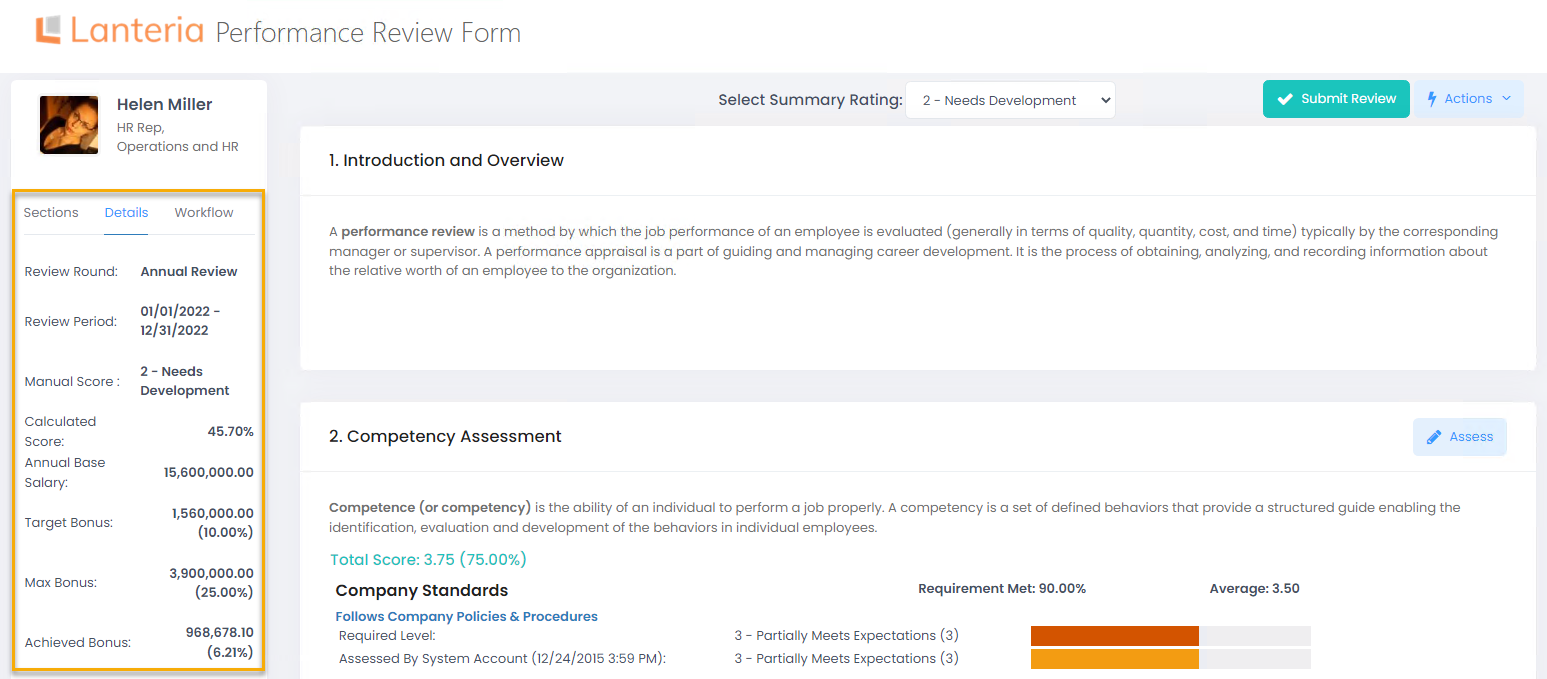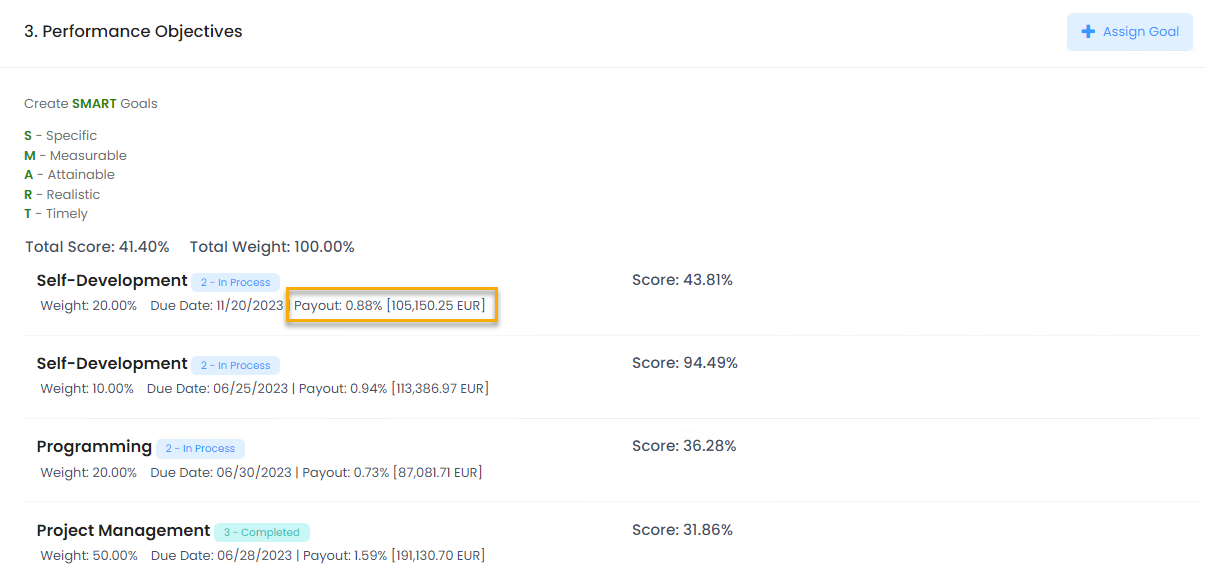• Lanteria HR
Bonus Calculation
• 25 Apr 2023
• Dark
Light

# Bonus Calculation

• Dark
Light

Article Summary

Based on the performance review results, the system will calculate the bonus the employee is entitled to.

For the bonus to be calculated, the following conditions must be met:

• The Calculate Bonus check box must be selected when starting a review round
• Job grades must be created under Compensation > Ranges and Grades or in the Compensation section of Settings and Configuration
• Bonus calculation rules must be created for the job grades on the Settings and Configuration panel under Compensation > Advanced > Bonus Calculation Rules or Compensation > Ranges and Grades > Grades. The bonus calculation rules define the target bonus percent (percent of the base salary employee will get in case of good performance) and the maximum bonus percent that the employee will get in case of the outstanding performance. The rules are set up for and linked to job grades.

## Calculation Process

The part of the target bonus the employee is entitled to is calculated based on the employee's achievement of the personal objectives. The payout is calculated depending on the % Complete or rating of each goal and the goal weight. Payout calculation also depends on the type of goal (qualitative or quantitative) and, in case of quantitative goals, on the Best Value setting of the quantitative goal (More when  higher value is considered better or Less when lower value is considered better).

Note
Whether the payout and thus, bonus is calculated based on the goal percent complete or rating depends on whether the rating is specified. If the rating is assigned to a goal, it has priority over the  Percent Complete value.

The achieved bonus can be viewed on the Review Details tab of the performance review form.The payout for each qualitative goal is calculated as follows:

'Payout' = 'Goal Rating' * 'Goal Weight' * 'Target Bonus'

The payout for each quantitative goal is calculated as follows:

• For quantitative goals with Best Value set to More:
• If Actual Result is empty, then Payout = 0
• If Actual Result ≤ Threshold, then Payout = 0
• If Actual Result ≤ Target, then payout is calculated as follows:
'Payout' = ('Actual Result' - 'Threshold') / ('Target Result' - 'Threshold') * 'Goal Weight' * 'Target Bonus'
• If Target < Actual Result ≤ Max Result, then payout is calculated as follows:
'Payout' = (1 + ('Actual Result' - 'Target Result') / ('Max Result' - 'Target Result') * ('Max Bonus' - 'Target Bonus') / 'Target Bonus') *'Goal Weight' * 'Target Bonus'
• If Actual Result > Max Result, then payout is calculated as follows:
'Payout' = (1 + ('Max Result' - 'Target Result') / ('Max Result' - 'TargetResult') * ('Max Bonus' - 'Target Bonus') / 'Target Bonus')  *'Goal Weight' * 'Target Bonus'
• For quantitative goals with Best Value set to Less:
• If Actual Result is empty, then Payout = 0
• If Actual Result ≥ Threshold, then Payout = 0
• If Actual Result ≥ Target, then payout is calculated as follows:
'Payout' = ('Threshold' - 'Actual Result') / ( 'Threshold' - 'Target Result') * 'Goal Weight' * 'Target Bonus'
• If Actual Result >= Max Result, then payout is calculated as follows:
'Payout' = (1 + ('Target Result' - 'Actual Result') / ('Target Result' - 'Max Result') * ('Max Bonus' - 'Target Bonus') / 'Target Bonus') *'Goal Weight' * 'Target Bonus'
• if Actual Result < Max Result, then payout is calculated as follows:
'Payout' = (1 + ('Target Result' - 'Max Result') / ('Target Result' - 'Max Result') * ('Max Bonus' - 'Target Bonus') / 'Target Bonus') *'Goal Weight' * 'Target Bonus'
Note
If the actual value is more than maximum value, the maximum value and not the actual result is used in the calculation.

Next, the achieved bonus for each objective is calculated as Payout * Annual Base Salary. You can see the bonus amount in the employee salary currency next to each objective in the review form.

The summary for all the objective amounts will comprise the Achieved Bonus amount (that is, which part of the target bonus has been achieved).